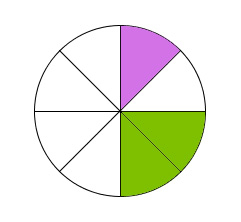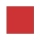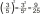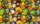# Simplify 7

Simplify. 7-1/3÷ 3-2/3 of 2+ 4-1/2÷ 2-1/4+ 1/2 solution and by step by step

x =  9.5556

### Step-by-step explanation:Did you find an error or inaccuracy? Feel free to write us. Thank you!Tips to related online calculators
Need help to calculate sum, simplify or multiply fractions? Try our fraction calculator.

## Related math problems and questions:

• Simplify 3Simplify mixed numerals expression: 8 1/4- 3 2/5 - (2 1/3 - 1/4) Show your solution.
• Sum of fractionsWhat is the sum of 2/3+3/5?
• Fractions and mixed numerals(a) Convert the following mixed numbers to improper fractions. i. 3 5/8 ii. 7 7/6 (b) Convert the following improper fraction to mixed number. i. 13/4 ii. 78/5 (c) Simplify these fractions to their lowest terms. i. 36/42 ii. 27/45 2. evaluate following ex
• SimplifySimplify powers multiplication: (3+22)(5-42)
• Simplify expression with mixedWhen (7¹/3 + 2¾)÷(2 + 2¼ × 1¹/3) is simplified the result is?3 3/4 + 2 3/5 + 5 1/2 Show your solution.
• Factors 2A number has 3 identical factors. If 1 of them is 18. Find the number step by stepTo three-eighths of one third, we add five quarters of one half and multiply the sum by four. How much will we get?
• Evaluate 12Evaluate and simplify 9 divided by 7/6.
• Brackets 2Add parenthesis to make true: 5-2×6-4+2=5
• CandiesThere are 150 candies in the bowl. Ivan took 7 thirtieths, Charles 6 fifteenths, and Thomas 3 tenths. How many sweets are left in the bowl?
• Complicated sum minus productWhat must be subtracted from the sum of 3/8 and 5/16 to get difference equal to the product of 5/8 and 3/16?
• Simplify:Simplify: 8(−9 − 5x) + 4b use x = 9 and b = −9
• Length subtractingExpress in mm: 5 3/10 cm - 2/5 mm
• Evaluate fractionsThe difference  of 5 1/2 and 2/3 is added to the product of 5/6 and 1/2
• When 5When 5 is subtracted from both my numerator and denominator I become 3/4. What fraction i am?
• Quotient and productIf the quotient of [8/5 divided by 8/10] is added to the product of [8/14 x 7/12 x 3/8], what is the sum?